# CS 415 Programming Languages Fortran Aaron Bloomfield Fall

• Slides: 25
Download presentationCS 415: Programming Languages Fortran Aaron Bloomfield Fall 2005 1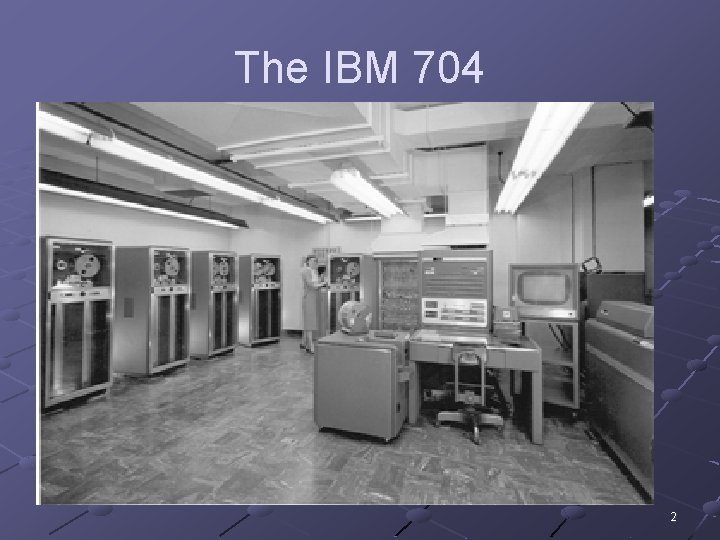The IBM 704 2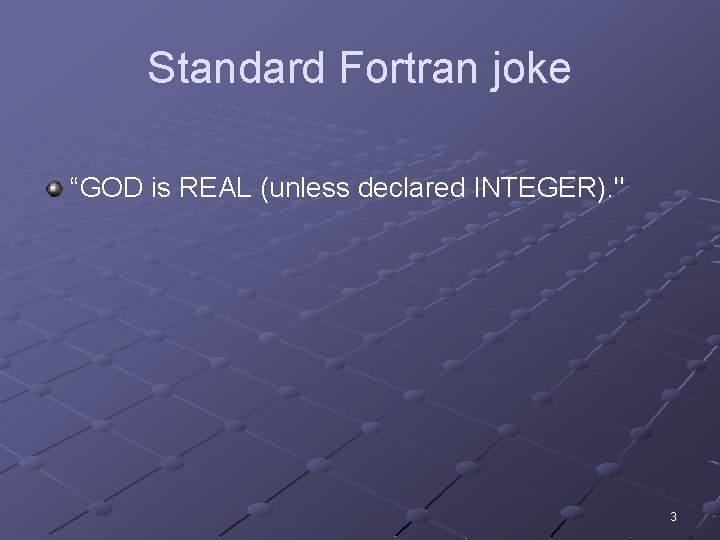Standard Fortran joke “GOD is REAL (unless declared INTEGER). " 3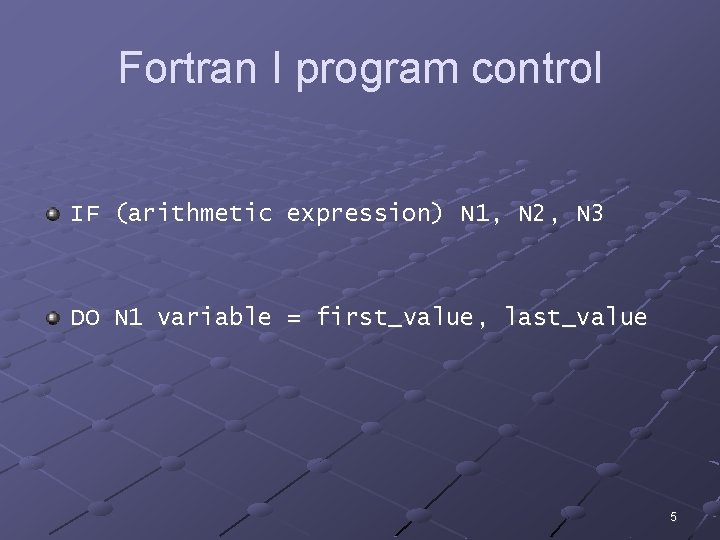Fortran I program control IF (arithmetic expression) N 1, N 2, N 3 DO N 1 variable = first_value, last_value 5Punch cards 6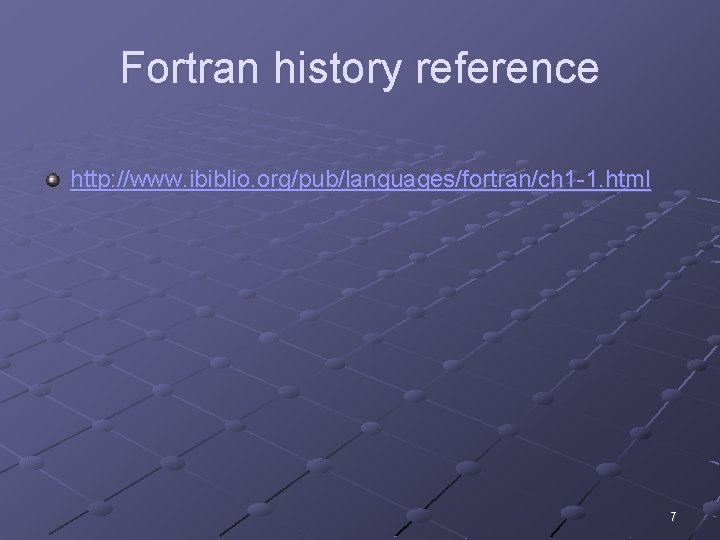Fortran history reference http: //www. ibiblio. org/pub/languages/fortran/ch 1 -1. html 7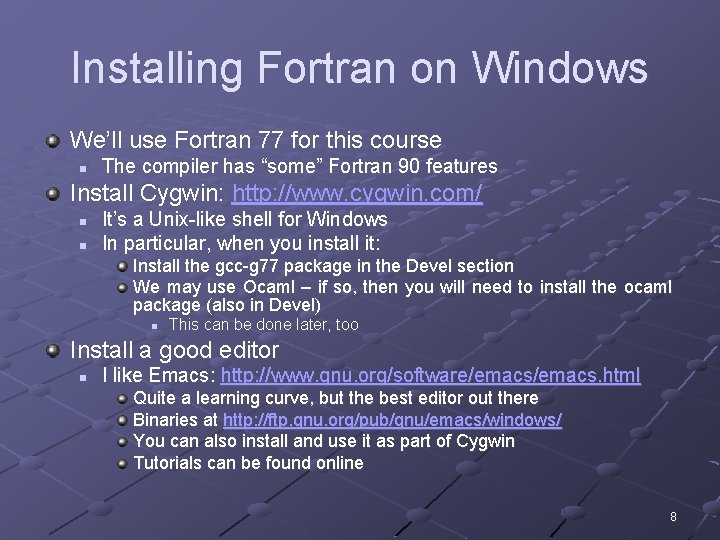Installing Fortran on Windows We’ll use Fortran 77 for this course n The compiler has “some” Fortran 90 features Install Cygwin: http: //www. cygwin. com/ n n It’s a Unix-like shell for Windows In particular, when you install it: Install the gcc-g 77 package in the Devel section We may use Ocaml – if so, then you will need to install the ocaml package (also in Devel) n This can be done later, too Install a good editor n I like Emacs: http: //www. gnu. org/software/emacs. html Quite a learning curve, but the best editor out there Binaries at http: //ftp. gnu. org/pub/gnu/emacs/windows/ You can also install and use it as part of Cygwin Tutorials can be found online 8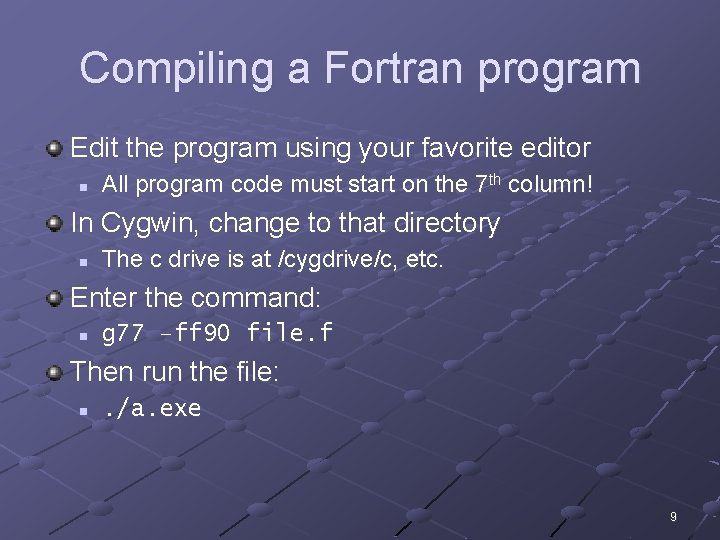Compiling a Fortran program Edit the program using your favorite editor n All program code must start on the 7 th column! In Cygwin, change to that directory n The c drive is at /cygdrive/c, etc. Enter the command: n g 77 –ff 90 file. f Then run the file: n . /a. exe 9Hello world in Fortran PROGRAM Hello. World PRINT *, 'Hello world!' END PROGRAM Hello. World Column 1 Column 7 10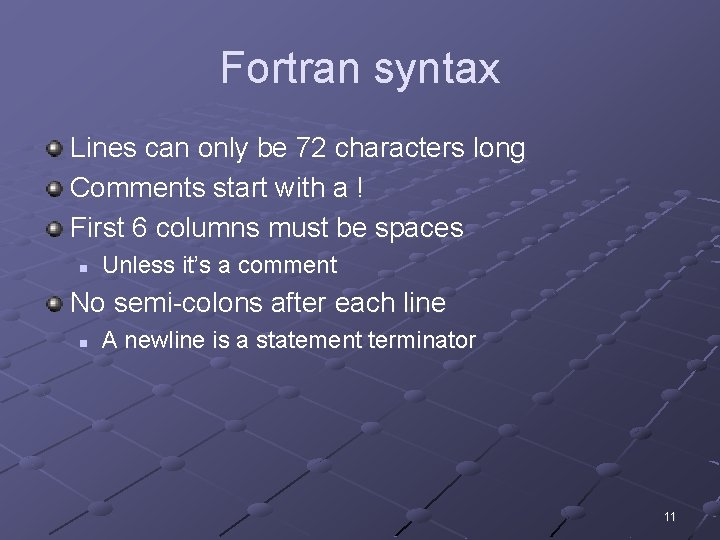Fortran syntax Lines can only be 72 characters long Comments start with a ! First 6 columns must be spaces n Unless it’s a comment No semi-colons after each line n A newline is a statement terminator 11Variable declaration The types are real, integer, etc. real : : x, y, z integer : : a, b, c character : : g Always put ‘implicit none’ at the beginning n n Right after the ‘program’ line Prevents implicit variable declaration 12Input and output Output statement: n print *, "(", tri 1 x, ", ", tri 1 y, ")“ Input statement: n read *, tri 3 x There are ways to do nicely formatted output n We aren’t going over them 13Operators Boolean operators: . and. , . or. , . not. , etc. n n Basically the name of the operation in periods Boolean values are. true. and. false. Relational operators: <, >, <=, >=, ==, /= 14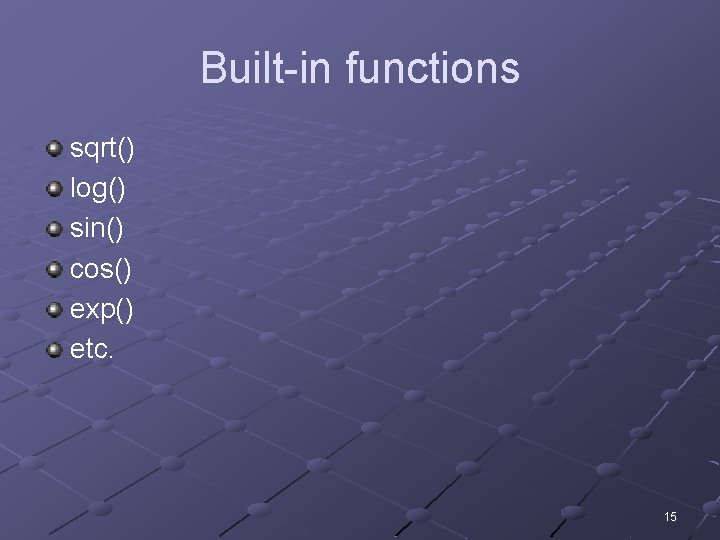Built-in functions sqrt() log() sin() cos() exp() etc. 15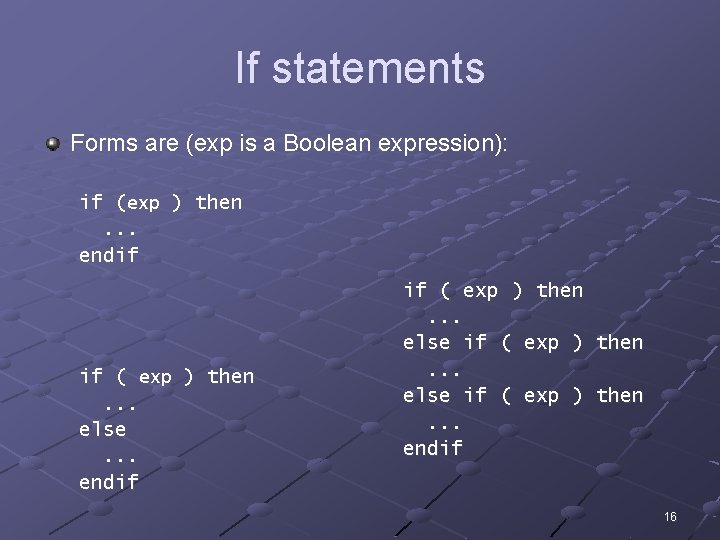If statements Forms are (exp is a Boolean expression): if (exp ) then. . . endif if ( exp ) then. . . else if ( exp ) then. . . endif 16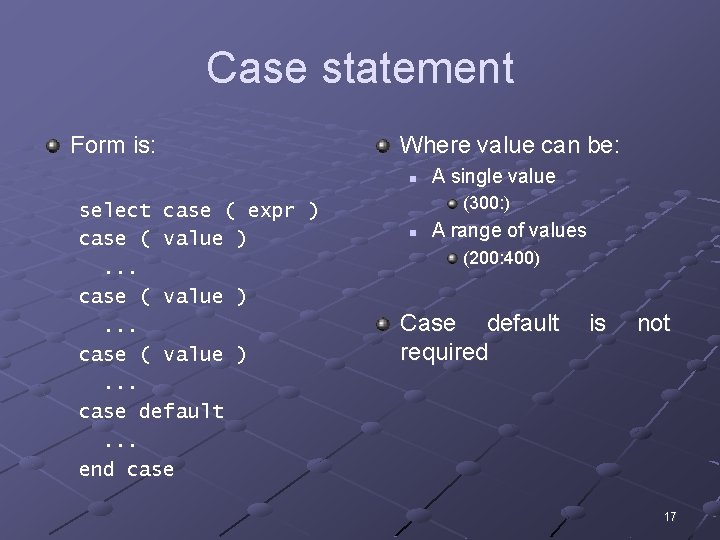Case statement Form is: Where value can be: n select case ( expr ) case ( value ). . . case default. . . end case A single value (300: ) n A range of values (200: 400) Case default required is not 17Looping Form is: do i = 1, 10. . . end do do i = 1, 10, 2. . . end do The first loops from 1 to 10 The second loops from 1 to 10, but odd numbers only 18Loop control Exit n Exits the loop, not the program Cycle n n Similar to next or continue in other languages Starts the next iteration of the loop 19Demo program Computes the sin, cos, tan, etc. ! This program allows the user to input the number of ! and then computes the cosine, and tangent. It ! user inputs "n" or "N". PROGRAM angle IMPLICIT none ! Type variables. REAL : : cosine, tangent, degrees REAL : : pi = 3. 141592 CHARACTER : : choice DO ! Enter and read the number of degrees in the angle. PRINT *, "Enter the number of degrees in the READ *, degrees ! Convert number of degrees in angle to radians. degrees = degrees*(pi/180) ! Use intrinsic functions to compute values. cosine=cos(degrees ) cosine=cos(degrees) sine=sin(degrees) tangent=tan(degrees) ! Print results. PRINT *, "cosine=", cosine, " sine=", sine, " ! Give user chance to exit program. PRINT *, "Would you like to do this again? " PRINT *, "(Press n to exit - any other key to READ *, choice ! Exit loop if the value in choice is N or n. IF (choice == "N". or. choice == "n") EXIT END DO STOP END PROGRAM angle degrees in an angle continues until the angle. " tangent=", tangent continue. )" 20Demo program Computes the average ! This program averages a series of numbers input ! from the keyboard. PROGRAM average IMPLICIT none ! Type variables. REAL : : data, sum, avg INTEGER num, i ! Prompt for and enter number of numbers to average. PRINT *, "Enter the number of numbers to average. " READ *, num sum = 0. 0 ! Loop goes from 1 to number of values to average. DO i = 1, num ! Prompt for and enter a number. PRINT *, "Enter a value for the number" READ *, data ! Add number to total. sum = sum + data END DO ! Calculate average. avg = sum/real(num) ! Print results. PRINT *, "The average = ", avg STOP END 21Demo program Computes the average via a defined function ! This program uses a function to find the average of three numbers. PROGRAM func_ave ! Type variables in main program (a, b, and c are local variables). REAL : : a, b, c, average ! Prompt for and get numbers to be averaged. PRINT *, "Enter the three numbers to be averaged. " READ *, a, b, c ! Invoke function average PRINT *, "The three numbers to be averaged are ", a, b, c PRINT *, "The average of the three numbers is ", average(a, b, c) STOP END PROGRAM func_ave ! Function average REAL FUNCTION average(x, y, z) ! Type variables in function (x, y, and z are local varialbes). REAL : : x, y, z ! Function name contains the average the function calculates and returns. average = (x + y + z)/3. 0 RETURN END FUNCTION average 22Fortran gotchas All variables must be declared at the beginning Remember line limit of 72 characters! n Consider: integer : : first, second, third, fourth, fifth, sixth, seventh, eighth, nin ni n n The 8 th variable is named ‘ei’ There is no 9 th variable declared Column 72! No continuation lines in Fortran 77 == is comparison for if's Can’t seem to be able to change the values of parameters in functions 23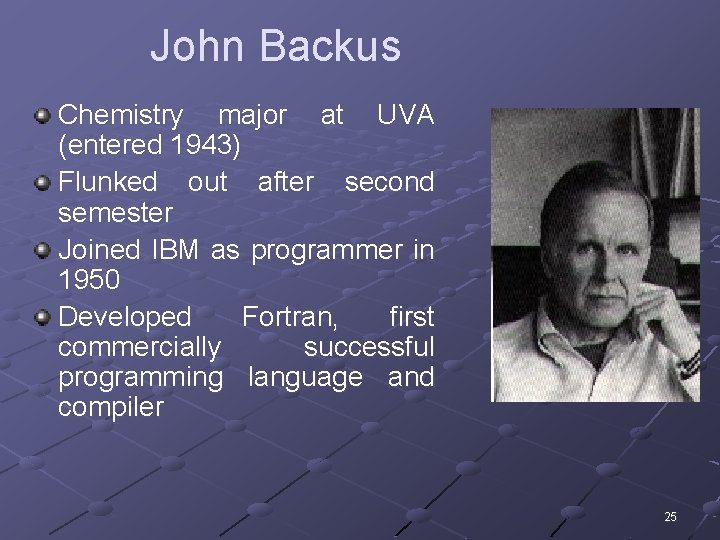John Backus Chemistry major at UVA (entered 1943) Flunked out after second semester Joined IBM as programmer in 1950 Developed Fortran, first commercially successful programming language and compiler 25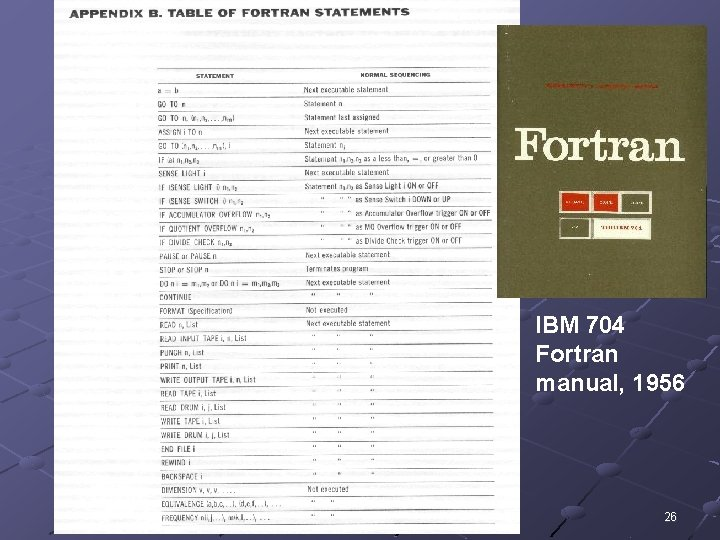IBM 704 Fortran manual, 1956 26Fortran issues… Fortran language was described using English n n n Imprecise Verbose, lots to read Ad hoc DO 10 I=1. 10 Assigns 1. 10 to the variable DO 10 I Early Fortrans didn’t care about spaces! DO 10 I=1, 10 Loops for I = 1 to 10 (Often incorrectly blamed for loss of Mariner-I) 27